# Closed Captioned Video: Algebra Applications: Exponential Functions, Segment 3: What Is the Difference between Earthquake Intensity and Magnitude?

In this episode of Algebra Applications, students explore earthquakes using exponential models. In particular, students analyze the earthquake that struck the Sichuan Province in China in 2008, months before the Beijing Olympics. This dramatic, real-world example allows students to apply their understanding of exponential functions and their inverses, along with data analysis and periodic function analysis.

## —PRESS PREVIEW TO SEE THE VIDEO—

This is part of a collection of videos from the Algebra Applications video series on the topic of Exponential Functions.

Below is a review of exponents and exponential functions. This review may be helpful before reviewing the Algebra Applications videos.

### Brief Review of Exponents

When a number is raised to a power, exponents are involved. Here are the key components of an exponential expression.

Exponents make it easier to write expressions that would otherwise be cumbersome to write. See how much easier it is to write 310 instead of the expression on the right?Count the number of 3's in the expression on the right. Do you see that there are ten 3's? The expression 310 is a shorthand way of writing 3 multiplied ten times.

In the expression below, 3 is called the base and 10 is called the exponent. The base is multiplied by itself 10 times.

### 310

Exponents are a different form of writing numbers. With this new form come new properties that are unique to exponents. The next section goes over these properties, also known as the Laws of Exponents.

### Laws of Exponents

The Laws of Exponents are a set of properties that pertain to all exponential expressions. Study the definition below.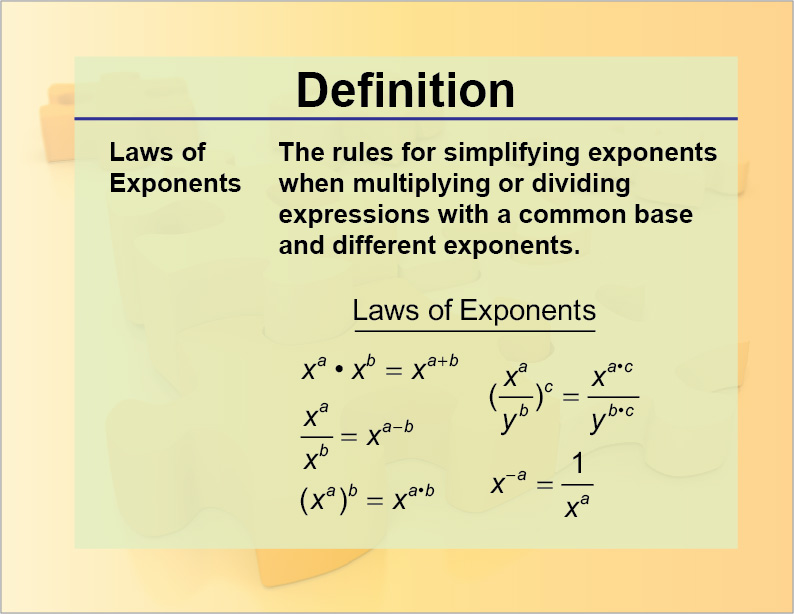To learn more about exponents, click on this link. It is a presentation that goes over the Laws of Exponents in more detail. To see examples that use the Laws of Exponents, click on this link

In summary:

• If you're multiplying two exponents that have the same base, then simply add the exponents.
• If you're dividing two exponents that have the same base, then subtract the exponents.
• If you're raising an exponential expression to a power, the new exponent is the product of the two exponents.

 Exponential Expressions Example 1 What is the value of x?In order to work with the Laws of Exponents, make sure the terms have the same base. Both 8 and 32 can be written as powers of 2:Rewrite the original expression with these powers of 2:With a common base, you can now solve for x:Example 2 If 4x - 5y = 10, what is the value of this expression?Find a common base for the numerator and denominator, then simplify the expression:Do you notice that the exponent for 2 is the same as the left side of the linear equation? Replace the exponentsExample 3 Rewrite this expression as an exponential expression with a power of 5.First, write the numerical expression as an exponential expression:Next, rewrite 125 as a power of 5:Finally, rewrite the entire expression:### Exponential Functions

An exponential function is of this form:

### f(x) = a•bcx

In this function the terms a, b, and c are numbers and x is the independent variable. Here’s one of the simplest exponential functions:

### f(x) = 2x

This is the graph of the function.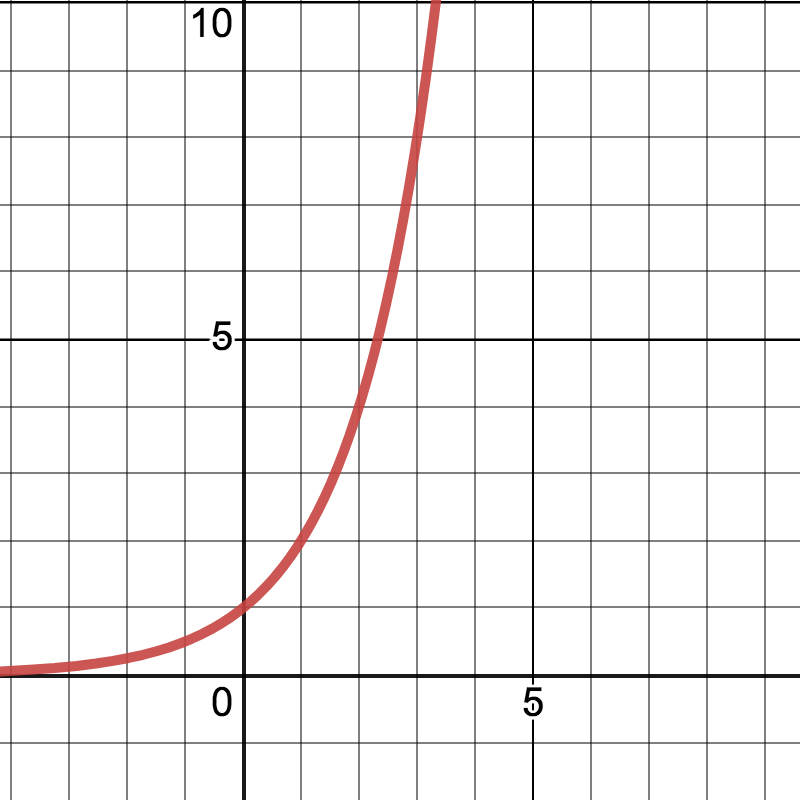As you can see from the graph, the values of f(x) increase dramatically for small increases in input values of x. Here are some other examples of exponential functions.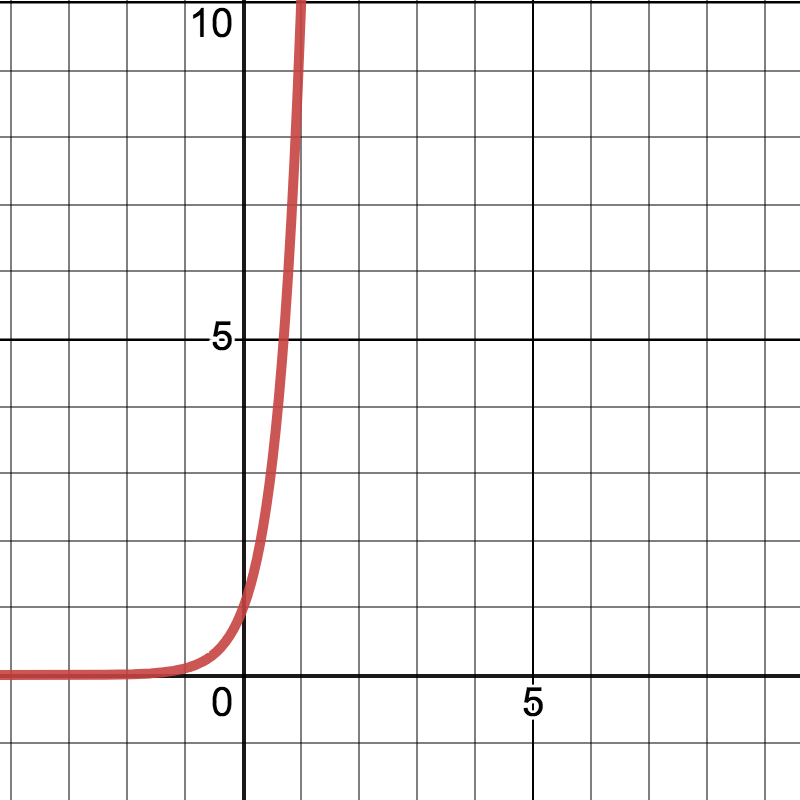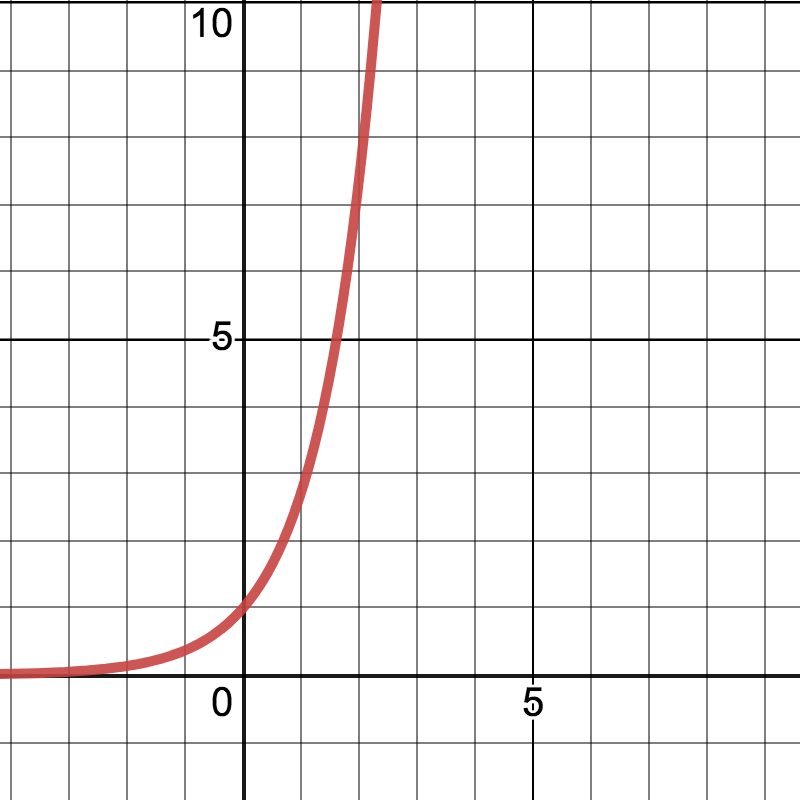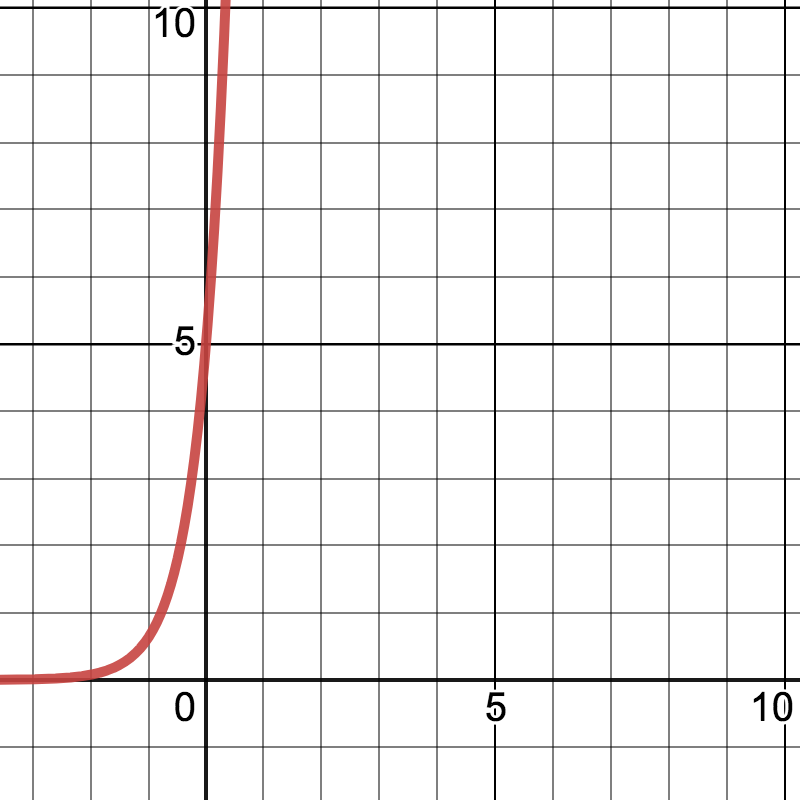### Exponential Growth and Decay

In the examples you saw previously, the graphs of the exponential functions were continuously increasing. But this isn't always the case.

When the the graph of an exponential function is continuously increasing, This is an example of exponential growth. Here is an example.

f(x) = 10xWhen the the graph of an exponential function is continuously decreasing, This is an example of exponential decay. Here is an example.

f(x) = 10-x### Exponential Models

Mathematical functions are often used to develop mathematical models of real-world phenomena. For example, linear functions are useful for modeling constant speed, and many other applications. Quadratic functions can be used to model the motion of objects in the air due to the influence of gravity.

Why is it important to develop mathematical models using equations? One of the best features of an accurate mathematical model is that it can be used to make predictions about future behavior about the phenomenon that it models. In fact, scientists, engineers, and other STEM-related jobs rely heavily on mathematical models to predict weather, financial results, and many, many other phenomena.

In the case of exponential functions, how can they be used to model something? Any phenomenon that involves exponential growth and decay can be modeled using an exponential function. Here are some possible uses of exponential models:

• Radioactive decay can be modeled by an exponential function that exhibits exponential decay
• Compound interest (see below) can be modeled by an exponential function that exhibits exponential growth

### Building an Exponential Model: Compound Interest

A special case involving exponential functions is compound interest. What is compound interest? Usually, the context is of money earning interest in a bank account.

If you put an amount of money P in a bank account, then compound interest means that the value of P increases over time. The variable P is an abbreviation for the Principle, or original amount, that you're saving.

The exponential model for compound interest begins with P. Let f(x) stand for the future value of the money in the savings account.

f(x) = P • (Exponential Term)

In the case of compound interest, the Exponential Term is the exponential growth term. Let's use the variable G to stand for growth. The function now looks like this:

f(x) = P • Gnt

The exponent, nt, is made up of two terms. The n stands for the number of compounding periods, or the number of times the principal has grown in value. The t stands for the duration of a compounding period.

There are two formulas that can be used to calculate compound interest. Here is one of the compound interest formulas: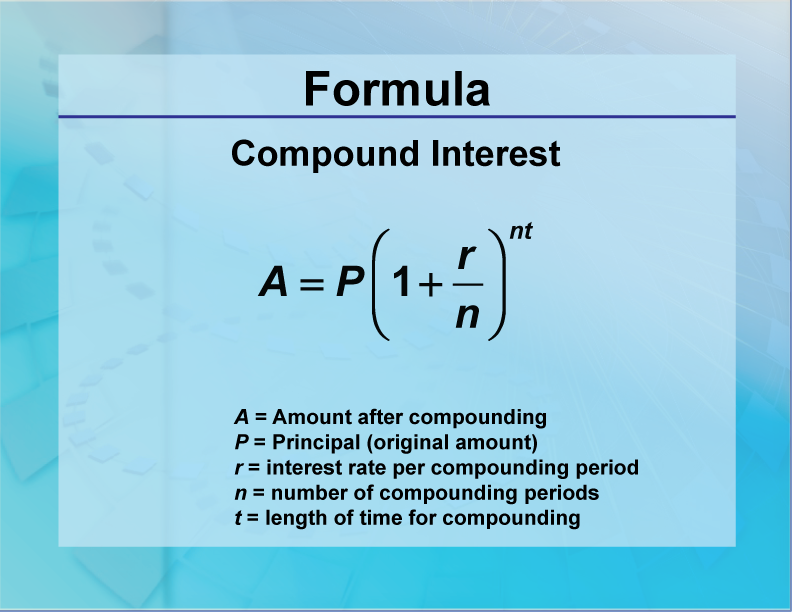This is the formula to use when the compounding periods are non-continuous. Use this formula for continuous compounding: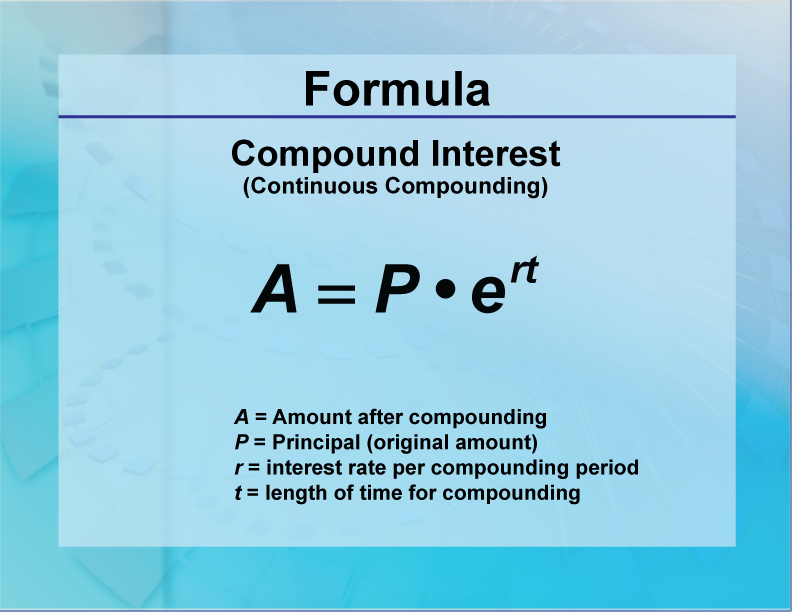To see examples of calculating compound interest, click on this link

 Compound Interest Example 1 A savings account earns interest according the formula below. What is the exponential function that models this growth? How much is in the savings account after five years? “Each year the amount of the account increases by 2.5%. The initial investment was \$1750.” Use the compound interest placeholder formula:In this formula: A = Current amount in savings P = Initial investment r = Interest rate n = Number of compounding periods t = Number of years We know the following: P = 1750 r = 0.025 n = 1 We can now generate the exponential function that models the growth:We can use this model to find the amount in savings after 5 years:### Inverse of an Exponential Function

Like many functions, exponential functions have inverses. The inverse of an exponential function is known as a logarithmic function.

This is the form of a logarithmic function:

f(x) = loga(x)

Here is the graph of a logarithmic function:Notice that, unlike an exponential function, a logarithmic function grows slowly.

Here is the graph of the exponential function that is the inverse of the logarithmic function.Notice that the graph of the logarithmic function (the red graph) is the mirror image of the exponential graph (the blue graph). This is a property of inverse functions. They are mirror images of each other across the graph of y = x. Imagine a mirror along the graph of y = x. Each function is a mirror image of the other across this line.

The logarithmic function f(x) = log (x) is "log base 10." It is the inverse of f(x) = 10x. Here are other exponential functions and their corresponding logarithmic inverses:

f(x) = log2(x) is the inverse of f(x) = 2x

f(x) = log3(x) is the inverse of f(x) = 3x

There is a special logarithm, known as the natural logarithm, whose base is the irrational number e. A natural log is written this way:

f(x) = ln(x)

The natural logarithm is the inverse of f(x) = ex. Here are the graphs of the tow functions.Notice that, like the other pair of exponential graphs, the two graphs are mirror images of each other.

Natural logs and exponential graphs of base e occur often in nature, thus the name "natural." These types of exponential functions are used to model many real-world phenomena.

## Video Transcripts

### This video has a transcript available. To see the complete collection of video transcripts, click on this link.

Common Core Standards CCSS.Math.CONTENT.HSF.LE.A.2 5.93 minutes 6 - 9 Algebra     • Exponential and Logarithmic Functions         • Applications of Exponential and Logarithmic Functions         • Exponential and Logarithmic Functions and Equations         • Graphs of Exponential and Logarithmic Functions 2020 algebra, applications of exponential functions, earthquakes, logarithms, magnitude of an earthquake, Closed Captioned Video